From puzzle to study of reality | Статья в сборнике международной научной конференции

Отправьте статью сегодня! Журнал выйдет 6 ноября, печатный экземпляр отправим 10 ноября.

Опубликовать статью в журнале
Проекты
Меню

Автор:

Рубрика: 9. Педагогика высшей профессиональной школы

Опубликовано в

V международная научная конференция «Педагогическое мастерство» (Москва, ноябрь 2014)

Дата публикации: 23.09.2014

Статья просмотрена: 6 раз

### Библиографическое описание:

Рутман, Яков. From puzzle to study of reality / Яков Рутман. — Текст : непосредственный // Педагогическое мастерство : материалы V Междунар. науч. конф. (г. Москва, ноябрь 2014 г.). — Москва : Буки-Веди, 2014. — С. 251-253. — URL: https://moluch.ru/conf/ped/archive/144/6188/ (дата обращения: 28.10.2021).

Introduction

Why do children like to make puzzles? Finding the answer to this question led us to write this article. The answer is it is interesting to see how individual, uncoordinated pieces fit into one image. At first, the child needs help adults who will explain to him how it works. Further, the child learns and begins to act independently.

The same principle maintained in the process of study at school or university. People enjoy when they see as from separate pieces one can get one. It is an incentive, motivation for further study and learns new knowledge. It is necessary to develop a capacity for such vision of objects.

How the study of physics can help in the development of suchlike fundamental approach to things? We present here an example of how to combine scientific puzzle component parts, which are the themes of the various fields of physics. A complete description of the following phenomena can be found in Ref.1.

Main body

Circular motion is first identified in mechanics. Kinematics describes a circular motion, without going into details that is its cause. A body moving in a circle has a radial acceleration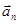. It is directed toward the center of the circle and its magnitude is determined by the formula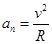, wherein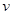- the body velocity,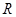-radius of the circle. Later, in the dynamics, determine the source of such a movement. It is a force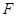that is directed racially towards the center. It holds the body in a circle. The equation of motion of the body around the circumference is as follows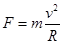. This equation is the basis of our puzzle.

Next we turn to the components of the puzzle. Chapter gravitation studies motion of a satellite. The trajectory of this movement is a circle, and the source is the force of attraction between the planet P and the satellite S. The equation of motion of the satellite looks like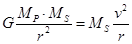(1)

where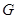- the universal gravitational constant,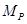- the mass of the planet,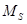- the mass of the satellite and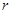- the distance between the centers of the planet and satellite. This is the first component of the puzzle. Represent it in the form of a picture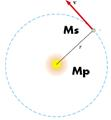Figure 1. Satellite.

After the mechanics students move to study the next chapter — «electricity». Coulomb's law defines a new force which describes the interaction between the electric charges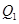and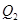located at a distanceeach from the other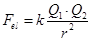.

In the «nuclear physics» the studying a structure of atom begins with a hydrogen atom. Semi-classical Bohr's model considers the motion of the electron around the nucleus (proton) in circular orbits. The equation of motion of the electron in the hydrogen atom is written as follows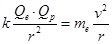(2)

where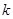— the electric constant,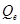and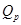— charge of an electron and a proton, respectively,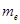— mass of an electron,- radius of the orbit of an electron in an atom. Consequently, the following picture of the puzzle looks like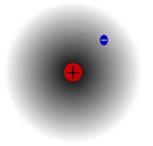Figure 2. The hydrogen atom

In the chapter «magnetism» there is a new force, the Lorentz force. This magnetic force acts on a moving charge in a magnetic field. The value of the Lorentz force is calculated as follows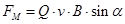, where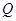— the charge of a particle,its velocity,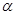the angle between the velocity and the magnetic field. If the magnetic field and the velocity of the particle perpendicular each to other, then particle trajectory is a circle, and its equation of motion is written as follows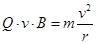(3)

where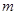- the mass of a charged particle. This result is used to create an accelerator of particles, the cyclotron. This last piece of the puzzle can be represented by a picture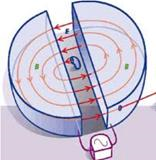Figure 3. Cyclotrons

Conclusion

The material presented in the article is studied in the course «General Physics». These courses for students of engineering specialties are taught during two years. The last few lectures of the course we dedicate a fundamental approach, which involves the study of relationships between different physical phenomena. This is an important aspect to have a positive impact on students' motivation.

In perspective we plan to create a course of lectures describing the connection between the phenomena from different fields of science. The task of the teacher is to teach the students to see how the individual pieces fit into a single unit. Here are some examples of drawing up such a «puzzles».

1)                 The equation of a simple harmonic oscillator describes the various physical phenomena: the oscillation mass on a spring; pendulum motion; motion of objects in a circle; the motion of charges in the electrical circuit LC; harmonic oscillator in quantum mechanics.

2)                 Accelerators, satellites and atoms as an example of circular motion.

3)                 Electric current and hydrodynamics.

References:

1.                           Serway R. A. and Jewett J. W.: Physics for Scientists and Engineers; 6th edition, Thomson Brooks/Cole; 2004